In :
from __future__ import print_function
from __future__ import division
import pandas as pd
import numpy as np
import datetime
import random

In :
import matplotlib.pyplot as plt
%matplotlib inline
from seaborn import set_style
set_style("darkgrid")
import seaborn as sns

/usr/local/lib/python2.7/site-packages/matplotlib/__init__.py:872: UserWarning: axes.color_cycle is deprecated and replaced with axes.prop_cycle; please use the latter.
warnings.warn(self.msg_depr % (key, alt_key))

In :
def build_features(features, data):
# remove NaNs
data.fillna(0, inplace=True)
data.loc[data.Open.isnull(), 'Open'] = 1
# Use some properties directly
features.extend(['Store', 'CompetitionDistance', 'Promo', 'Promo2', 'SchoolHoliday'])

# Label encode some features
features.extend(['StoreType', 'Assortment', 'StateHoliday'])
mappings = {'0':0, 'a':1, 'b':2, 'c':3, 'd':4}
data.StoreType.replace(mappings, inplace=True)
data.Assortment.replace(mappings, inplace=True)
data.StateHoliday.replace(mappings, inplace=True)

features.extend(['DayOfWeek', 'Month', 'Day', 'Year', 'WeekOfYear'])
data['Year'] = data.Date.dt.year
data['Month'] = data.Date.dt.month
data['Day'] = data.Date.dt.day
data['DayOfWeek'] = data.Date.dt.dayofweek
data['WeekOfYear'] = data.Date.dt.weekofyear

# CompetionOpen en PromoOpen from https://www.kaggle.com/ananya77041/rossmann-store-sales/randomforestpython/code
# Calculate time competition open time in months
features.append('CompetitionOpen')
data['CompetitionOpen'] = 12 * (data.Year - data.CompetitionOpenSinceYear) + \
(data.Month - data.CompetitionOpenSinceMonth)
# Promo open time in months
features.append('PromoOpen')
data['PromoOpen'] = 12 * (data.Year - data.Promo2SinceYear) + \
(data.WeekOfYear - data.Promo2SinceWeek) / 4.0
data['PromoOpen'] = data.PromoOpen.apply(lambda x: x if x > 0 else 0)
data.loc[data.Promo2SinceYear == 0, 'PromoOpen'] = 0

# Indicate that sales on that day are in promo interval
features.append('IsPromoMonth')
month2str = {1:'Jan', 2:'Feb', 3:'Mar', 4:'Apr', 5:'May', 6:'Jun', \
7:'Jul', 8:'Aug', 9:'Sept', 10:'Oct', 11:'Nov', 12:'Dec'}
data['monthStr'] = data.Month.map(month2str)
data.loc[data.PromoInterval == 0, 'PromoInterval'] = ''
data['IsPromoMonth'] = 0
for interval in data.PromoInterval.unique():
if interval != '':
for month in interval.split(','):
data.loc[(data.monthStr == month) & (data.PromoInterval == interval), 'IsPromoMonth'] = 1

return data

In :
print("Load the training, test and store data using pandas")
types = {'CompetitionOpenSinceYear': np.dtype(int),
'CompetitionOpenSinceMonth': np.dtype(int),
'StateHoliday': np.dtype(str),
'Promo2SinceWeek': np.dtype(int),
'SchoolHoliday': np.dtype(int),
'PromoInterval': np.dtype(str)}

Load the training, test and store data using pandas

In :
print("Assume store open, if not provided")
test.fillna(1, inplace=True)

# print("Consider only open stores for training. Closed stores wont count into the score.")
# train = train[train["Open"] != 0]
# print("Use only Sales bigger then zero")
# train = train[train["Sales"] > 0]

print("Join with store")
train = pd.merge(train, store, on='Store')
test = pd.merge(test, store, on='Store')

features = []

print("augment features")
train = build_features(features, train)
test = build_features([], test)
print(features)

print('training data processed')

Assume store open, if not provided
Join with store
augment features
['Store', 'CompetitionDistance', 'Promo', 'Promo2', 'SchoolHoliday', 'StoreType', 'Assortment', 'StateHoliday', 'DayOfWeek', 'Month', 'Day', 'Year', 'WeekOfYear', 'CompetitionOpen', 'PromoOpen', 'IsPromoMonth']
training data processed


What must be forecasted ? The sales per store. For what period ?

In :
print ('From',test.Date.min(),'to', test.Date.max())
print ('That is', test.Date.max()-test.Date.min(), 'days')

From 2015-08-01 00:00:00 to 2015-09-17 00:00:00
That is 47 days 00:00:00 days


For how many stores ?

In :
test.Store.nunique()

Out:
856

Let's take a random store from the trainings data and plot how the Sales data looks like

In :
rS = 979 # rS =  random.choice(train.Store.unique())
print ('Random store number =', rS)

Random store number = 979


How many year's of data do we have in the trainingset?

In :
train.Year.unique()

Out:
array([2013, 2014, 2015])

Let look at the sales of store 979 in 2013

In :
train[(train.Store==rS) & (train.Year==2013)].Sales.plot(label='2013', figsize=(18,4))
plt.title('Store {}'.format(rS))
plt.show()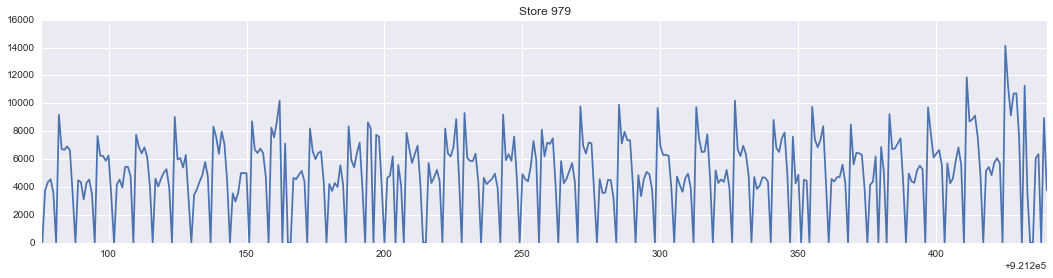We see some patters emerge. Let's make Date the index so that we have date's at the x-axis.

In :
train.set_index('Date', inplace=True)

In :
st = train[train.Store==rS] # Select store rS
st['2013']['Sales'].plot(label='2013', figsize=(18,4), title='Store {}'.format(rS))
plt.show()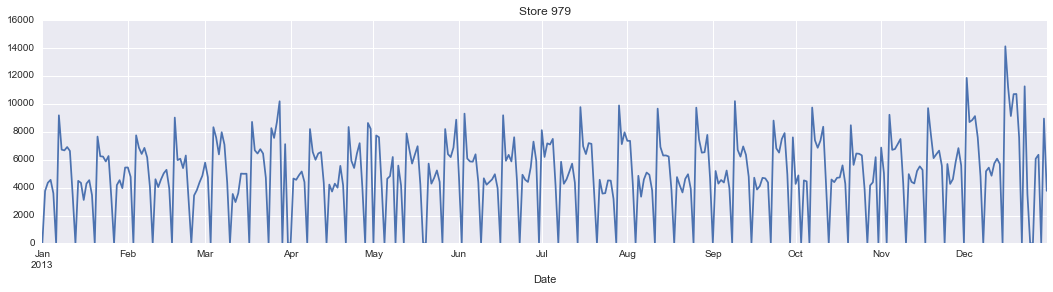The sharp needles in the Sales that touch the zero axis are the sunday's. The reason is that on Sunday most store are not open in Germany and have no sales. Let's check that by summing all sales on Sunday's:

In :
train[train.DayOfWeek==6].Sales.sum()

Out:
29551433.0

This should be zero. How come it's not? The reason is that some store's are occasionally open on sunday:

In :
salesOnSundayPerStore = train[(train.Open) & (train.DayOfWeek==6)].groupby('Store')['Sales']
salesOnSundayPerStore.count().sort_values().plot(kind='barh')
plt.title('Number of sunday open per store')
plt.show()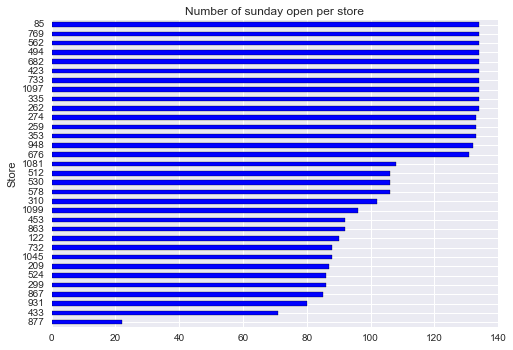Indeed, store number 85 had many open days on sunday:

In :
train[(train.Store==85) & (train.DayOfWeek==6)].Sales.plot(figsize=(18,4))
plt.title('Sales of store 85 on sundays')
plt.show()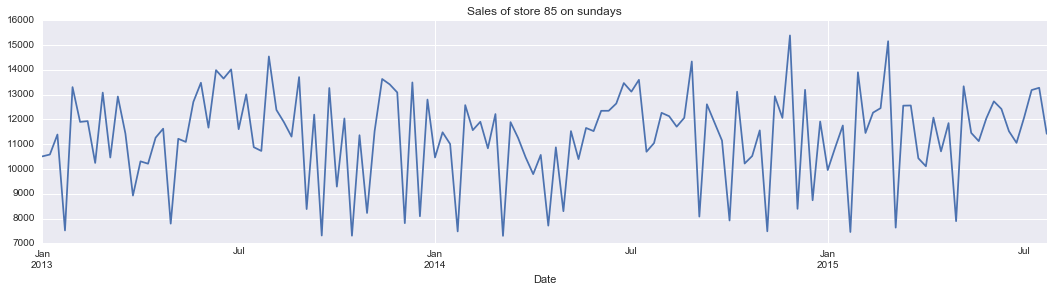Let's take a look to the sales of the store 979 and search for patterns.

In :
# fig, axes = plt.subplots(3, 1, figsize=(18, 4));
def plotStore(rS):
st = train[train.Store==rS]
storerS13 = st[st.Year==2013].Sales.reset_index(drop=True)
storerS14 = st[st.Year==2014].Sales.reset_index(drop=True)
storerS15 = st[st.Year==2015].Sales.reset_index(drop=True)

df_plot = pd.concat([storerS13, storerS14, storerS15], axis=1)
df_plot.columns = ['2013', '2014', '2015']
df_plot.index = pd.date_range('1/1/2015', periods=365, freq='D')
df_plot.plot(subplots=True,figsize=(18, 6), title='Sales at store {}'.format(rS))
plt.show()
plotStore(979)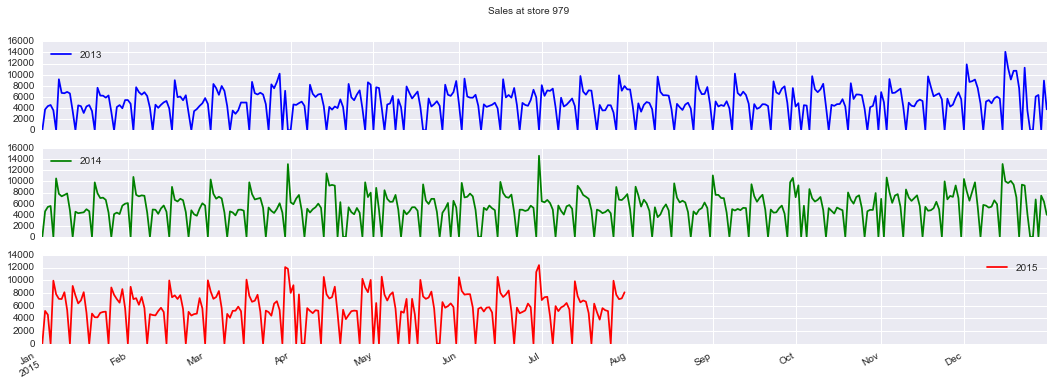From above chart, our task is clear. We have to predict how the red curve is continuing for 48 days starting from the first of august until and included 19 september. We can also spot some patterns. Peak's are the beginning of every month. The second week have rather constant sales. On the beginning of the third week, we see again peak altough a bit smaller than the beginning of the month. The reason for this patterns is probably paycheck days typically at the beginning of the month or in the middle of the month. Also in 2014 and 2015 we see a big peak in the beginning of July but not in 2013. Maybe a lot of Germans got extra holdiday money in 2014 and 2015 on there paycheck in July? Let's check another store.

In :
rS = 1013  # rS =  random.choice(train.Store.unique())
plotStore(1013)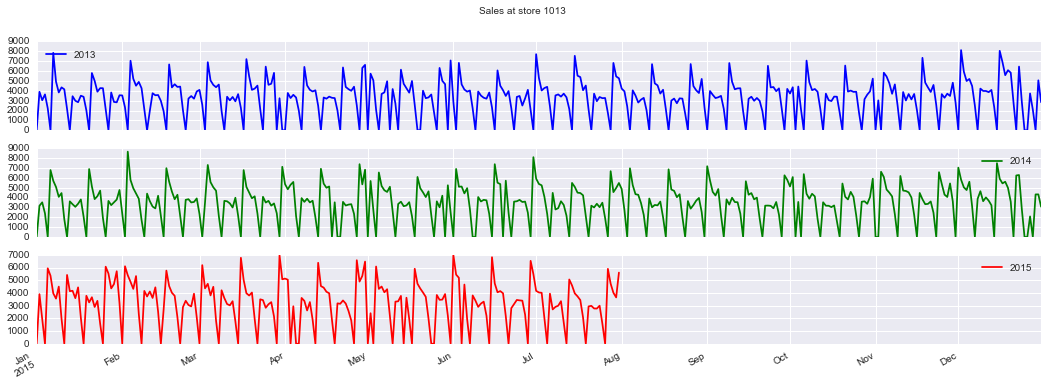Store 1013 has no extra big peak beginning of July. Let check another store.

In :
rS =  85 #random.choice(train.Store.unique())
plotStore(rS)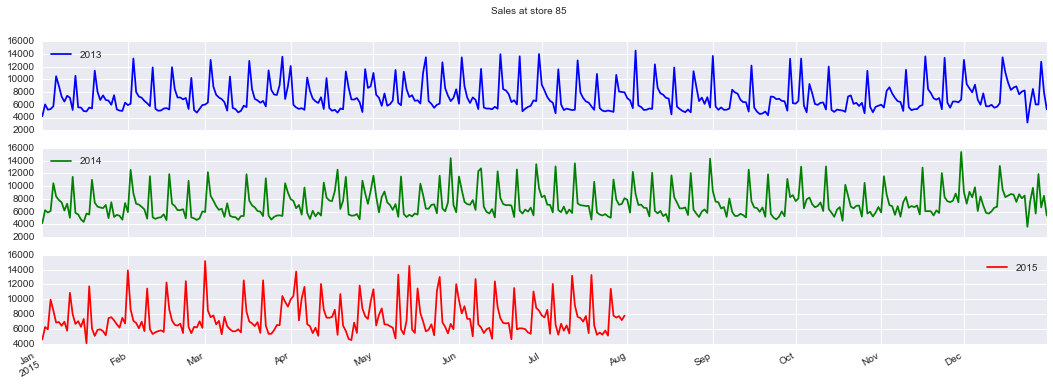Store 85 looks different. Remember store 85 ? It's the store that is open on sundays a lot. Let check another store that is open on sunday a lot: store 769.

In :
plotStore(769)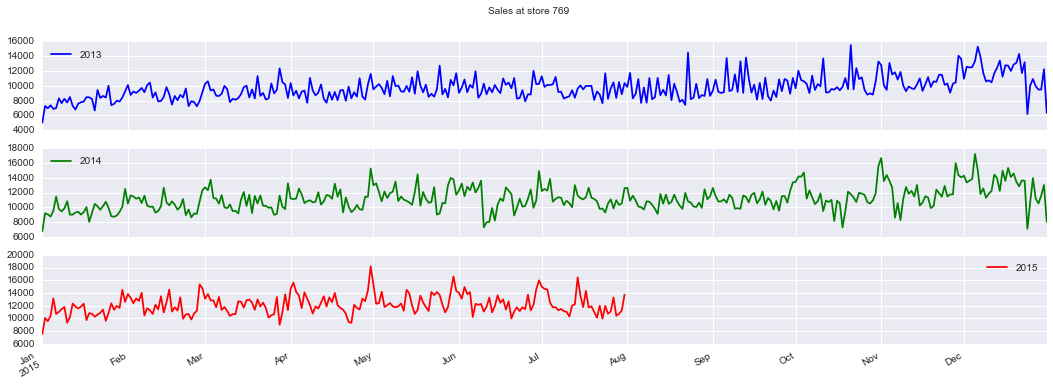We are lucky because neither store 86 nor store 769 are to be predicted so we can ingnore them. Still have to check for the other stores later.

# First Prediction¶

Sales look rather a constant repeating pattern. Let's exploit that pattern to make a prediction. The most basic assuption could be that sales of the store in same period but one or two year ago are a good prediction for this year.

Let's take the mean of August and the first two weeks of September in 2013 and 2014 as prediction:In :
trainStore = train[train.Store == rS]
prevy1 = trainStore.ix['2014-08-02':'2014-09-18']['Sales'].reset_index(drop=True)
prevy2 = trainStore.ix['2013-08-03':'2013-09-19']['Sales'].reset_index(drop=True)
meanSales = np.mean(np.vstack((prevy1, prevy2)), axis=0)
df_plot = pd.DataFrame(meanSales, index = pd.date_range('8/1/2015', periods=48, freq='D'))
df_plot.columns = ['Prediction']
df_plot.plot(title='Prediction for store {}'.format(rS));